# ELECTRIC POTENTIAL ENERGY ELECTRIC ENERGY ELECTRIC POTENTIAL V

• Slides: 16ELECTRIC POTENTIAL ENERGY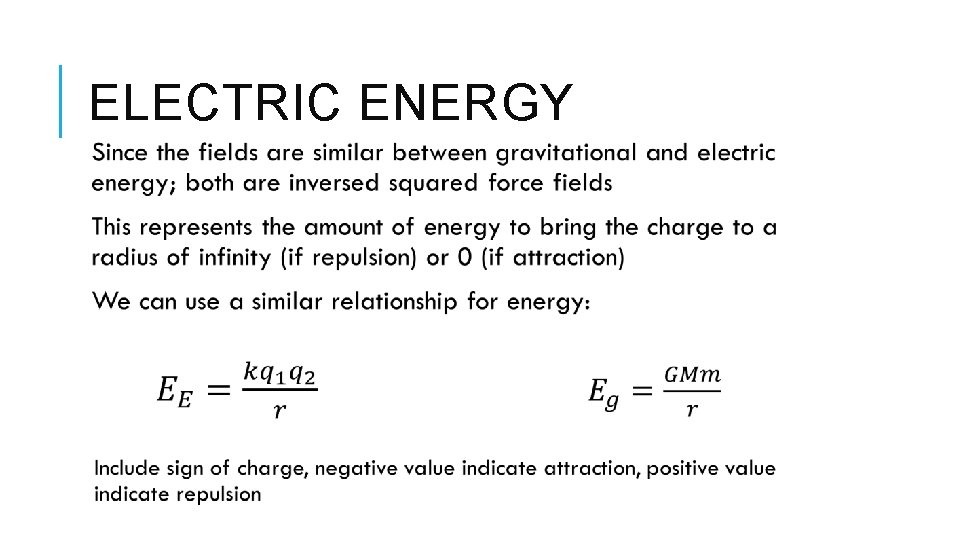ELECTRIC ENERGY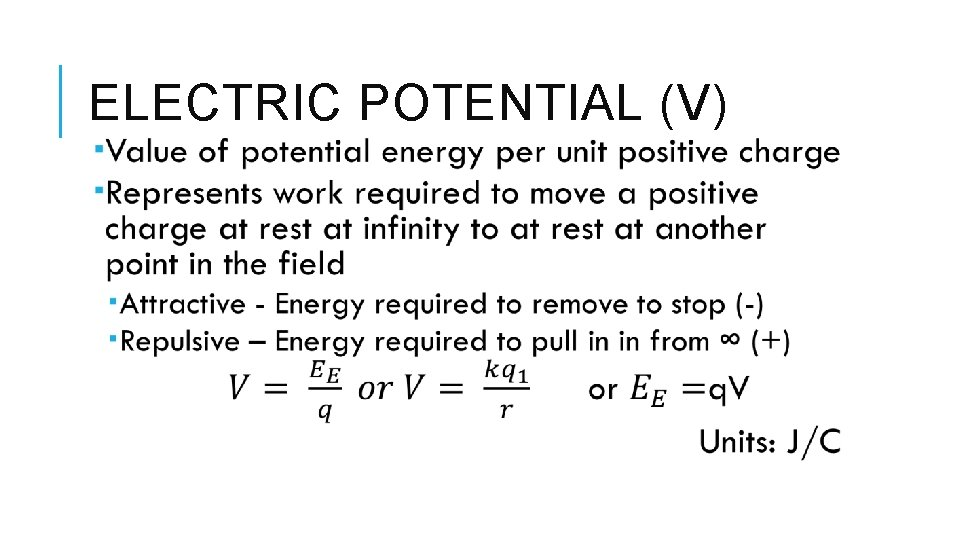ELECTRIC POTENTIAL (V)At r=o, Energy is large positive value Very close, lot’s of stored energy because it is ready to be pushed away with a lot of force At r= infinity, energy is small positive value, very little potential to be pushed At r=o, Energy is large negative value Very close, requires lot’s of external energy to be pushed away to infinity At r= infinity, energy is small negative value, very little energy required to be pushed further away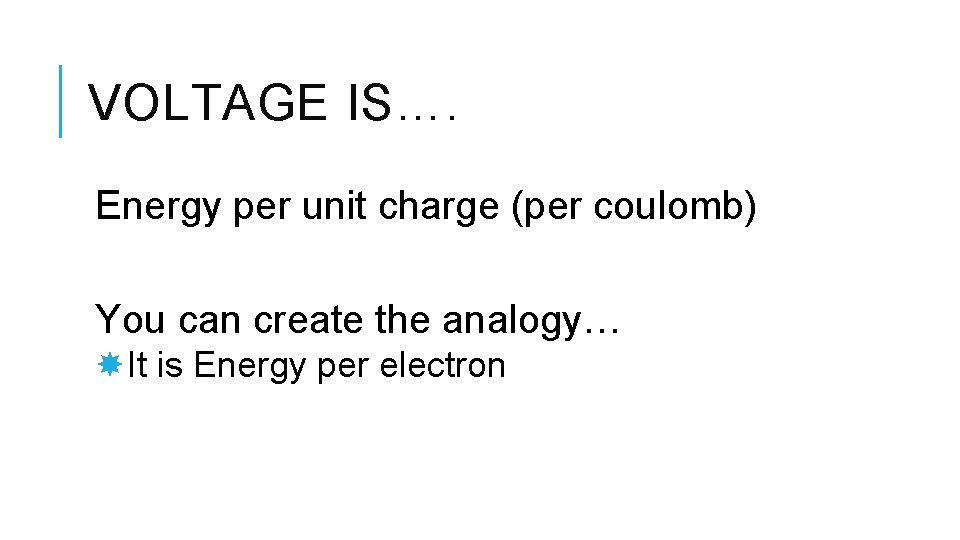VOLTAGE IS…. Energy per unit charge (per coulomb) You can create the analogy… It is Energy per electron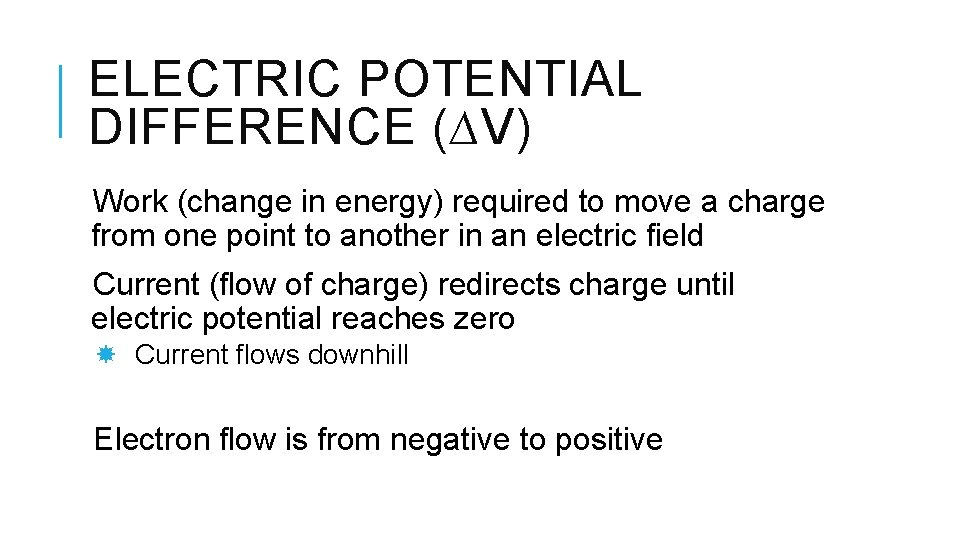ELECTRIC POTENTIAL DIFFERENCE (∆V) Work (change in energy) required to move a charge from one point to another in an electric field Current (flow of charge) redirects charge until electric potential reaches zero Current flows downhill Electron flow is from negative to positive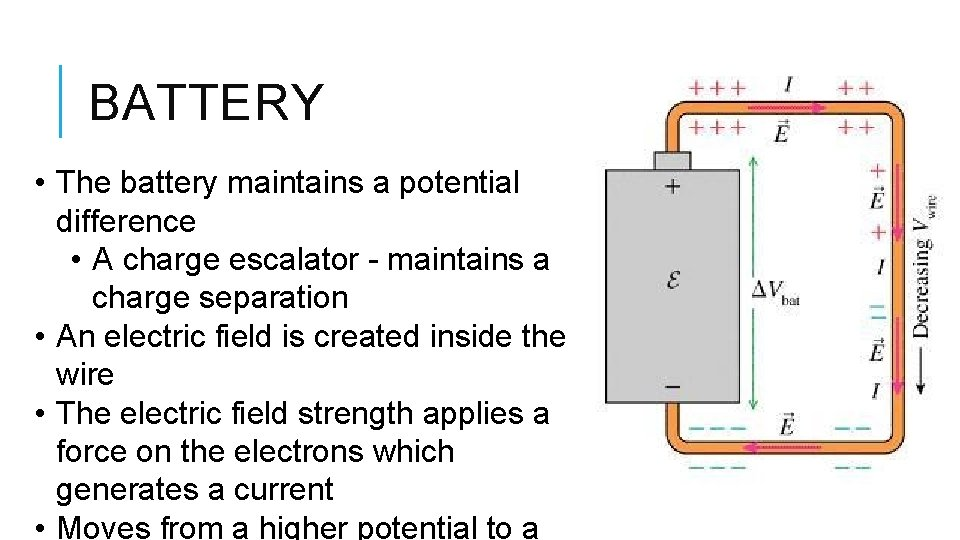BATTERY • The battery maintains a potential difference • A charge escalator - maintains a charge separation • An electric field is created inside the wire • The electric field strength applies a force on the electrons which generates a current • Moves from a higher potential to a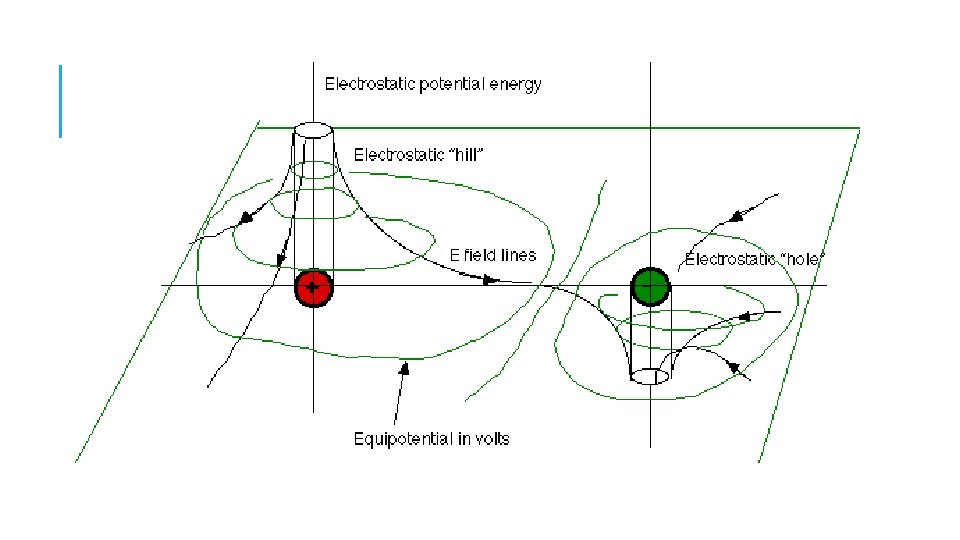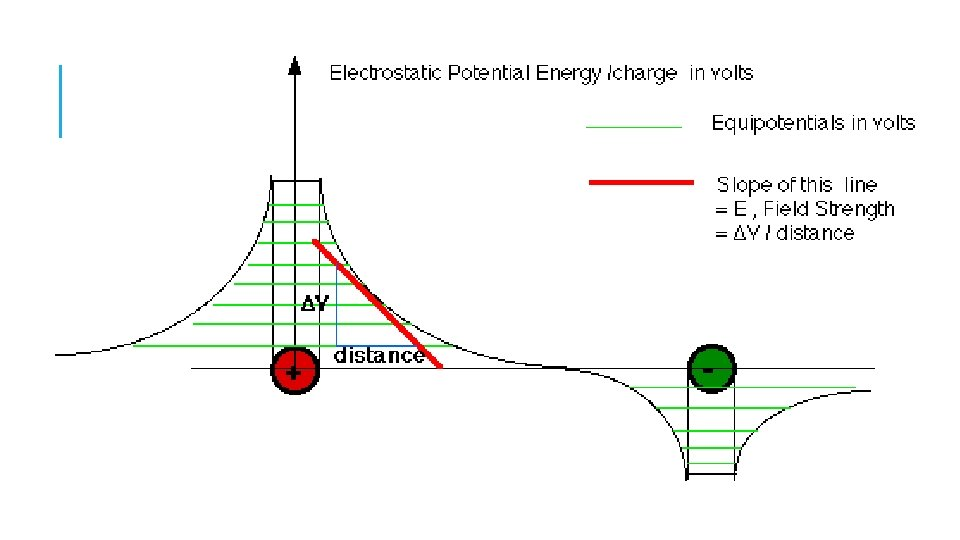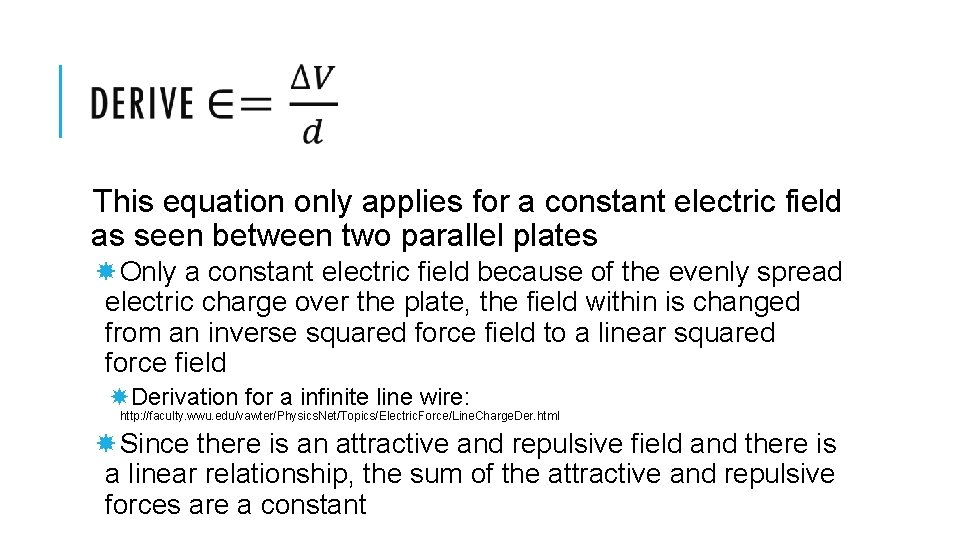This equation only applies for a constant electric field as seen between two parallel plates Only a constant electric field because of the evenly spread electric charge over the plate, the field within is changed from an inverse squared force field to a linear squared force field Derivation for a infinite line wire: http: //faculty. wwu. edu/vawter/Physics. Net/Topics/Electric. Force/Line. Charge. Der. html Since there is an attractive and repulsive field and there is a linear relationship, the sum of the attractive and repulsive forces are a constantApplies for a point charge in an electric fieldNOTE W = ∆Ee Work can also be used to produce kinetic energy as ∆Ee=∆Ek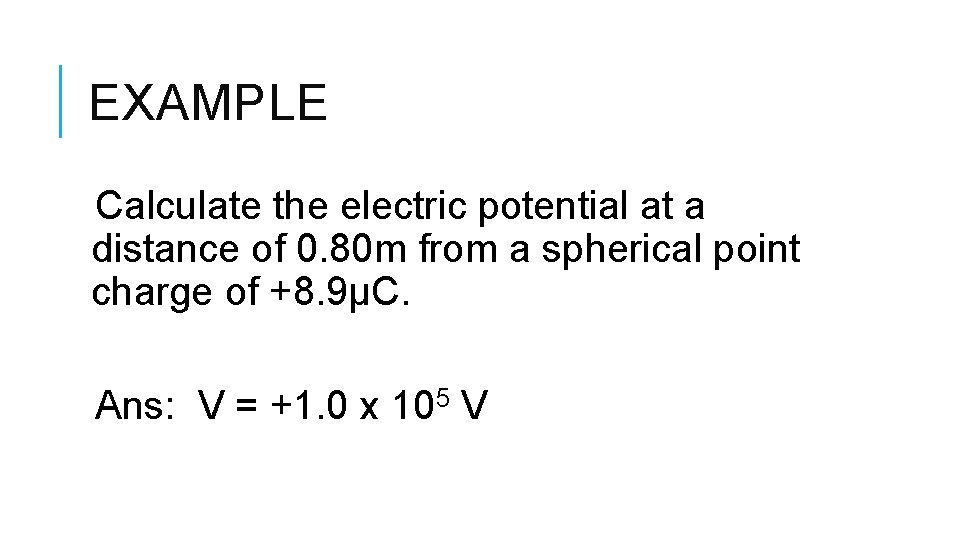EXAMPLE Calculate the electric potential at a distance of 0. 80 m from a spherical point charge of +8. 9µC. Ans: V = +1. 0 x 105 V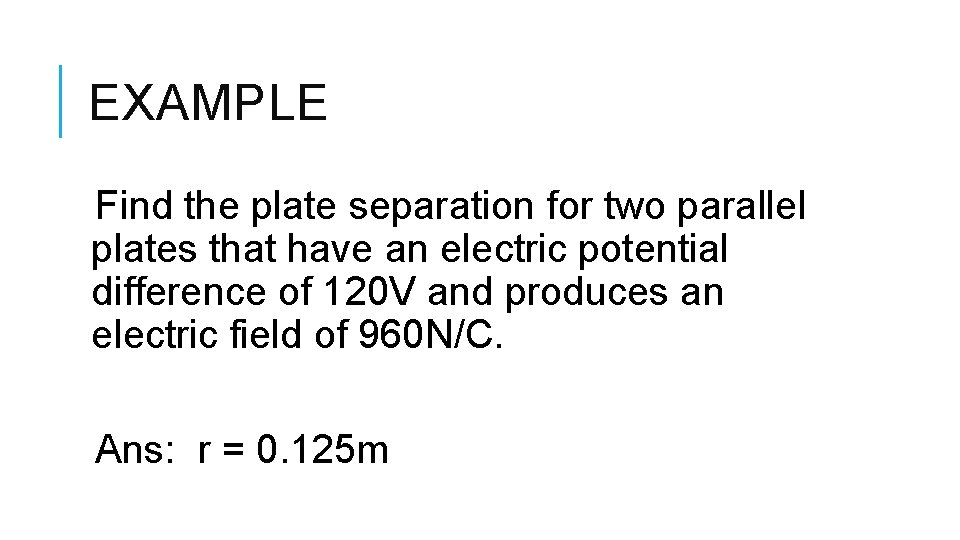EXAMPLE Find the plate separation for two parallel plates that have an electric potential difference of 120 V and produces an electric field of 960 N/C. Ans: r = 0. 125 mEXAMPLE How much work is done to increase the potential of a charge of 15µC with 120 V? Ans: W = 1. 8 x 10 -3 J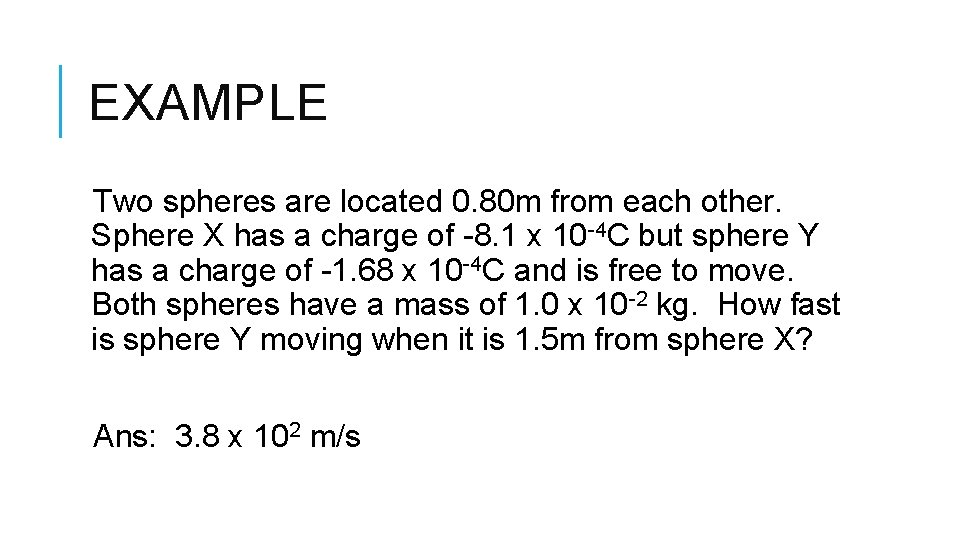EXAMPLE Two spheres are located 0. 80 m from each other. Sphere X has a charge of -8. 1 x 10 -4 C but sphere Y has a charge of -1. 68 x 10 -4 C and is free to move. Both spheres have a mass of 1. 0 x 10 -2 kg. How fast is sphere Y moving when it is 1. 5 m from sphere X? Ans: 3. 8 x 102 m/s